Do you like the article?
Share it with others -
Use new possibilities of MetaTrader 5

# Trend Lines Indicator Considering T. Demark's Approach

7 August 2008, 08:345
4 447

### Introduction

The struggle between buyers ("bears") and sellers ("bulls") can be demonstrated using trend lines. Thomas Demark developed the methods of objective choosing two points for TD-line of the trend drawing (you can find the detailed information about T. Demark's approach to technical analysis here).

Since, according to T. Demark, the most important thing for a trader is the last state of the market, in the suggested version of the indicator the last direction of the trend is drawn with a thick solid line, and it won't be odd for the analysis of the current state to know the nearest preceding direction of the trend, which is drawn with a thin dotted line.

### Some Features of the MQL4 Code of the Suggested Indicator

Four buffers are used in the indicator. Two buffers are for the code of arrows of lines direction and two of them are for the elongation of TD-line to the left side.

The first ten bars (to the left of the second TD-point) are drawn by the indicator's buffers in the «aqua» color.

```#property indicator_chart_window
#property indicator_buffers 4
#property indicator_color1 Chartreuse
#property indicator_color2 Tomato
#property indicator_color3 Aqua
#property indicator_color4 Aqua

// ----- indicator_buffers
double   TrendUpBuffer[];
double   TrendDownBuffer[];
double   TrendLineBufferUp[];
double   TrendLineBufferDown[];```

Initialize the functions that control the calculation and the indicator visualization.

```int init()
{
//---- indicators
SetIndexStyle(0,DRAW_ARROW);        // the type and the style of the indicator line
SetIndexArrow(0,236);               // the icon for the indicator line
SetIndexBuffer(0,TrendUpBuffer);    // the declaration of the unidimensional array
SetIndexEmptyValue(0,0.0);          // the size of the empty value of the indicator
SetIndexStyle(1,DRAW_ARROW);
SetIndexArrow(1,238);
SetIndexBuffer(1,TrendDownBuffer);
SetIndexEmptyValue(1,0.0);
SetIndexStyle(2,DRAW_LINE);
SetIndexBuffer(2,TrendLineBufferUp);    // for the rising line
SetIndexEmptyValue(2,0.0);
SetIndexStyle(3,DRAW_LINE);
SetIndexBuffer(3,TrendLineBufferDown);  // for the descending line
SetIndexEmptyValue(3,0.0);
//----
return(0);```

The deinitialization can be left empty by default.

```int deinit()
{
//  DelObj1();
//  DelObj2();
return(0);
}```

Create the function for deleting the object having the specified name.

```  void DelObj1()
{
ObjectDelete("TrDdown"); // Deleting of the object with the specified name.
}
void DelObj2()
{
ObjectDelete("TrDup");
}```

We'll search for the check points (for convenience) in separate cycles for peaks and troughs. We start the search from the 2nd bar, because we'll need to compare it with the right (non-zero) bar to clarify the verity of the trend line.

Let's check condition #1 (the price of the Max bar must be greater than the prices of the right and the left bar).

```   for(int i=2;i<48;i++) // 48 hours - 2 days  // i- index of the bar to be verified
{
if(High[i]>High[i+1]&&High[i]>High[i-1])
{```

Let's check condition #2 (the supporting price maximum should be higher than the close price beyond two bars before the registration).

In case the fulfillment of conditions #1 and #2 coincide, we place the counter of matches.

```      if(High[i]>Close[i+2])
{
U++; // the counter of matches of the 1st and the 2nd conditions
if(U==1)
{```

Then we pass to the by-peaks registration of the First check point parameters:

```         // The price of the First check point when the conditions #1 and #2 are fulfilled
TD_up=High[i];
index_up_1=i;                               // the index of the First check point
Pr_up_Cl_1=Close[i-1]; // the close price of the bar that follows the check point
time_up_1=iTime(NULL,0,index_up_1);          // the time of the first check point```

Here we can insert the following verifying code to control the program running:

```         // this is an easy-to-follow time to control the operators processing
Time_up_1=TimeToStr(time_up_1,TIME_DATE|TIME_MINUTES);
Print("  Up price of First check point = ",TD_up,"  ;  index = ",
index_up_1,"  time = ",Time_up_1);```

Since the counter has triggered once, we discover the second point in the further iterations and pass to the by-peaks registration of the Second check point parameters.

```          }
if(U==2)
{
// The price of the Second check point when the conditions #1 and #2 are fulfilled
TD_up=High[i];
index_up_2=i;            // the index of the Second check point
time_up_2=iTime(NULL,0,index_up_2);// the time of the Second check point```

Here we can insert the following verifying code to control the program running:

```           Time_up_2=TimeToStr(time_up_2,TIME_DATE|TIME_MINUTES);
Print("  Up price of Second check point = ",TD_up,"  ;  index = ",
index_up_2,"  time = ",Time_up_2);```

Now we have two TD-check points, we need to check the condition of the downtrend, i.e., the price of the First check point must be less than that of the Second check point.

```           // the condition of the downtrend (the right TD-point must be lower than the left one)
if((U==2 && TD_up<TD_up)==false)
{
Print(" Up the conditions of the downtrend are not fulfilled ");
U--; TD_up=0.0; index_up_2=0; time_up_2=0;  continue;
}```

If the condition is not fulfilled, we decrease the counter by one and zeroize the values of the price, index, time and return to the beginning of the cycle, in order to search for another Second check point. If the condition is fulfilled, we calculate the speed of TD-line falling.

```            else
{
Print(" Up the conditions of the downtrend are fulfilled ");
// the calculation of the speed of TD_max falling by two discovered points
V_up=(TD_up-TD_up)/(index_up_2-index_up_1);//speed(pips/bar)
// the calculated value of TD-line on the first bars which is to the right of Max
Pr_Tr_up_1=TD_up-V_up;
// if we subtract the product of speed of TD-line falling times number of bars
// from the price of the 1-st check point, we'll obtain the price of the
Pr_Tr_up_0=TD_up-(index_up_1*V_up);           // downtrend on the "0" bar```

Now let's check condition #3 (for the last supporting price maximum the close price for the next (to the right) bar must be lower than the calculated value of the speed of TD-line falling) – it is the condition of verity of the downtrend.

```              // let's check the condition #3 (for the last supporting price maximum the close
// price for the next (to the right) bar must be lower than the calculated
// value of the speed of TD-line falling)
if((Pr_up_Cl_1< Pr_Tr_up_1)==false)
{
Print(" Up The condition of verity of downtrend is not fulfilled!");
i=index_up_1+2; TD_up=0.0; TD_up=0.0; time_up_1=0;
time_up_2=0; index_up_1=50; index_up_2=50; U=0; continue;
}```

If condition #3 of verity of the downtrend is not fulfilled, then the search for Td-points should be begun again. To do it, it's necessary to zeroise the values of all variables obtained earlier, and begin the search from the bar that is to the left by 2 bars from the first found value of the First check point.

If the condition is fulfilled we memorize the values of calculated variables for drawing the trend line.

```             else
{ // if the condition #3 is fulffiled, the trend is true
Print("  Up the conditions of Downtrend verity are fulfilled ");
// memorize the values of variables for drawing the TD_Down line
TDu1=TD_up;  // price of the 1-st point
T_u1=time_up_1; // time of the 1-st point
TDu2=TD_up;  // price of the 2-nd point
T_u2=time_up_2; // price of the 2-nd point
TrendDownBuffer[index_up_1]=TDu1+5*Point;// put the down arrow```

Now let's fill the buffer with 10 values of the trend line to the left of the Second check point:

```                // prices of 10 bars of the trend line to the left of TD-point
for(int k=index_up_2;k<index_up_2+10;k++)
TrendLineBufferDown[k]=Pr_Tr_up_0+k*V_up;
Print("  exit from the cycle of searching, because 2 TD-points are found");
break;
}```

Insert the missing curly braces.

```               }
}
}
}
//==================================================================================+```

If the condition of verity is fulfilled, the trend line will be drawn to the left of the Second check point for 10 bars. Now this line is to be drawn to the right using the ObjectCreate() function. For this purpose the end of the program contains a block of trend lines drawing.

In this block, the first two operators control the thickness of the TD-line (STYLE_SOLID) involving the only one trend line on this stage. We draw the Descending line and exit from the cycle of searching the peaks for TD-points, preliminary deleting the previously existed line.

Using the above mentioned reasoning we write up the code for the rising line.

```//==================================================================================+
// for Min points (troughs)
for(int j=2;j<48;j++) // 48 hours - 2 days  // j- index of the bar to be checked
{
// let's check the conditon #1 (the price of the bar must be lower than the prices of the left and the right bars)
if(Low[j]<Low[j+1]&&Low[j]<Low[j-1])
{
// let's check the condition #2 (The supporting price Minimum must be lower than
// the close price of the bar beyond two bars before the registration <to the left of Min>)
if(Low[j]<Close[j+2])
{
D++;  // the counter of coincidences of the 1-st and the 2-nd conditions
if(D==1)
{
TD_down=Low[j];
index_down_1=j;
Pr_down_Cl_1=Close[j-1];
time_down_1=iTime(NULL,0,j);

Time_down_1=TimeToStr(time_down_1,TIME_DATE|TIME_MINUTES);
Print(" D price of the First check point ",TD_down," ; index ",index_down_1,
" ; Close to the right ",Pr_down_Cl_1,"  ; ",Time_down_1);
}
if(D==2)
{
TD_down=Low[j];
index_down_2=j;
time_down_2=iTime(NULL,0,j);

Time_down_2=TimeToStr(time_down_2,TIME_DATE|TIME_MINUTES);
Print(" D price of the Second check point ",TD_down," index ",index_down_2,
"  time ",Time_down_2);
// the condition of the rising trend (the right Min point must be higher than the left one)
if((D==2 && TD_down>TD_down)==false)
{
Print(" D The conditions of the Rising trend are not fulfilled! ");
D--;   TD_down=0.0;  continue;
}
else
{
Print(" D the conditions of the Rising trend are fulfilled");
// the calculation of the speed of TD_min rising by two discovered points
V_down=(TD_down-TD_down)/(index_down_2-index_down_1);
// the calculated value of the line on the 1-st bar to the right of Min
Pr_Tr_down_1=TD_down+V_down;
}
// let's check the condition #3 (for the last (to the right) price minimum,
// the close price of the next bar must be higher than the calculated value
// of the speed of TD-line rising).
if((Pr_down_Cl_1> Pr_Tr_down_1)==false)
{
i=index_down_1+1; TD_down=0.0; TD_down=0.0; time_down_1=0;
time_down_2=0; index_down_1=50; index_down_2=50; D=0; continue;
}
else
{ // the condition #3 is fulfilled, the trend is true
Print("  D the conditions of Rising trend verity are fulfilled ");
TDd1=TD_down;
T_d1=time_down_1;
TDd2=TD_down;
T_d2=time_down_2;
// the calculated price of the trend line on the "0" bar
Pr_Tr_down_0=TD_down+(index_down_1*V_down);
TrendUpBuffer[index_down_1]=TDd2-2*Point; // put the up arrow
// the price of 10 bars of the trend line to the right of the Second check point
for(int n=index_down_2;n<index_down_2+10;n++)
TrendLineBufferUp[n]=Pr_Tr_down_0-n*V_down;
Print("  D exit the cycle of searching, because two TD-points are found ");
break;
}
}
}
}
}
}
// ----------------------------------------------------------------------------+ ```

The block of drawing the trend lines is developed so the youngest TD-line is drawn with the bold solid line (STYLE_SOLID), and the line that is previous to it but with the opposite direction, is drawn with a thin dotted line (STYLE_DOT).

The situation when the trend goes in one direction for a long time, may occur. At that, the Second TD-point of the contrary trend won't be found and the intermediate values of the price, time and index will be written to the variables. To make this intermidiate values not to affect the drawing of the trend line, you should insert the filter of line drawing conditions: for example, if the Second TD-line is not found, the value of the price will be set to "0", at the same time, the value of the index of the First point will be fixed, and if this value will appear to be less than the value of the First TD-point of the line of opposite direction, then the last trend line that has the true parameters can be drawn with the other style and color. That is why the time filter is specified. Take a look at the attached file.

```// ----------------------------------------------------------------------------+
// the drawing of the trend lines to the right of the Second check point
// ----------------------------------------------------------------------------+
if(TDd2==0.0)   index_down_1=50;
if(TDu2==0.0)   index_up_1=50;
else
{
Print("  TDd2 = ",TDd2,"   index_up_1 = ",index_up_1,"  >  index_down_1 = ",index_down_1);
Print("  Up We draw the Descending one and exit from the cycle of searching the peaks for the TD-points");
DelObj1(); // preliminary deleting the previously existed line
ObjectCreate("TrDdown",OBJ_TREND,0,T_u2,TDu2,T_u1,TDu1);
if(index_up_1>index_down_1)
{           // the previous direction of the trend is drawn with:
ObjectSet("TrDdown",OBJPROP_COLOR,Yellow);
ObjectSet("TrDdown",OBJPROP_WIDTH,1);  // thin line and
ObjectSet("TrDdown",OBJPROP_STYLE,STYLE_DOT);// dotted line
}
else
{                // the very last direction of the trend is drawn with:
ObjectSet("TrDdown",OBJPROP_COLOR,Yellow);
ObjectSet("TrDdown",OBJPROP_WIDTH,2);           // thicker line
ObjectSet("TrDdown",OBJPROP_STYLE,STYLE_SOLID); // solid line
}
}
// -----------------------------

if(TDd2==0.0)   index_down_1=50;
else
{
Print("  TDd1 = ",TDd1,"   index_up_1 = ",index_up_1,"  <  index_down_1 = ",index_down_1);
Print("  D We draw the Rising one and exit from the cycle of searching the troughs for TD-points");
DelObj2(); // preliminary deleting the previously existed line
ObjectCreate("TrDup",OBJ_TREND,0,T_d2,TDd2,T_d1,TDd1);
if(index_up_1<index_down_1)
{

ObjectSet("TrDup",OBJPROP_COLOR,Yellow);
ObjectSet("TrDup",OBJPROP_WIDTH,1);
ObjectSet("TrDup",OBJPROP_STYLE,STYLE_DOT);
}
else
{
ObjectSet("TrDup",OBJPROP_COLOR,Yellow);
ObjectSet("TrDup",OBJPROP_WIDTH,2);
ObjectSet("TrDup",OBJPROP_STYLE,STYLE_SOLID);
}
}
// ----------------------------------------------------------------------------+
return(0);
}```

Notation: almost all operators "Print(...)" serve for the visual controlling of program execution, so they can be "commented", the operators of the string (TimeToStr()) type are necessary for controlling, as well.

### Conclusion

I hope, the suggested indicator can be used not only by the apprentice traders, but also by the experienced ones too, both for trading on the market and for the analysis of the performed biddings.

Translated from Russian by MetaQuotes Software Corp.
Original article: https://www.mql5.com/ru/articles/1507

Attached files |
TL_by_Demark_v6.mq4 (13.44 KB)

#### Other articles by this author

Last comments | Go to discussion (5)| 8 Aug 2008 at 18:18

This is a great indicator, but is it possible for you to put in an alert when the trend changes?

Ed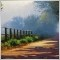| 17 May 2010 at 07:03

It is very good indictor,thank you ![Deleted] | 6 Jan 2011 at 22:40
It doesnt work with me. I drop it in a chart and don't activate (like there is something wrong). You know what's happening???| 5 Oct 2011 at 10:24

Congratulations on this fantastic indictor, i have paid out and tried so many, but yours is on real time, no lagging and yet accrate, i would like to make a donation to you, the only thing i think it needs is an alert.

Thank you for sharing.

God bless you.| 14 Jun 2014 at 08:04

Thank you for sharing.

It's really best indicator for me. :)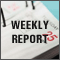All about Automated Trading Championship: Reporting the Championship 2006
This article contains Weekly Reports of the ATC 2006. These materials are like snapshots, they are interesting-to-read not only during the Championship, but years later as well.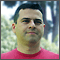All about Automated Trading Championship: Interviews with the Participants'06
Interviews with the Participants of Automated Trading Championship 2006 demonstrated a great variety of views of automated trading and trading as a whole. You can see yourselves which ideas turned out to work better during the Championship and which of them could not pass the critical verification through a three-month long "test-drive" on a contest account.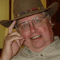All about Automated Trading Championship: Interviews with the Participants'07
The published interviews of Championship 2007 bear the stamp of the results obtained during the preceding contest. The first Championship evoked a wide response on the internet and in printings. The leading developer of the MetaQuotes Software Corp. tells about changes made to the forthcoming Automated Trading Championship 2007. We put our questions to the developer of a well-known indicating complex ZUP, Eugeni Neumoin (nen) and spoke to an equity trader, Alexander Pozdnishev (AlexSilver).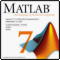Interaction between MetaTrader 4 and Matlab via DDE
Step-by-step instructions of how to organize data transfer from Matlab to MetaTrader 4 using DDE.